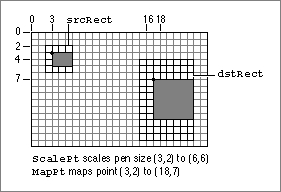# Legacy DocumentImportant: The information in this document is obsolete and should not be used for new development.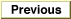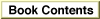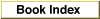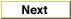Inside Macintosh: Imaging With QuickDraw /
Chapter 3 - QuickDraw Drawing / QuickDraw Drawing Reference
Routines / Scaling and Mapping Points, Rectangles, Polygons, and Regions

### ScalePt

To scale a height and width according to the proportions of two rectangles, use the `ScalePt` procedure.

```PROCEDURE ScalePt (VAR pt:\xDDPoint; srcRect,dstRect:\xDDRect);
```
`pt`
On input, an initial height and width (specified in the two fields of a `Point` record) to scale; upon completion, vertical and horizontal scaling factors derived by multiplying the height and width by ratios of the height and width of the rectangle in the `srcRect` parameter to the height and width of the rectangle in the `dstRect` parameter.
`srcRect`
A rectangle. The ratio of this rectangle's height to the height of the rectangle in the `dstRect` parameter provides the vertical scaling factor, and the ratio of this rectangle's width to the width of the rectangle in the `dstRect` parameter provides the horizontal scaling factor.
`dstRect`
A rectangle compared to the rectangle in the `srcRect` parameter to determine vertical and horizontal scaling factors.
##### DESCRIPTION
The `ScalePt` procedure produces horizontal and vertical scaling factors from the proportions of two rectangles. You can use `ScalePt`, for example, to scale the dimensions of the graphics pen.

You specify an initial height and width to scale in the `pt` parameter. This parameter is of type `Point`, although you don't pass coordinates in this parameter. Instead, you pass an initial height to scale in the `v` (or vertical) field of the `Point` record, and you pass an initial width to scale in the `h` (or horizontal) field.

The `ScalePt` procedure scales these measurements by multiplying the initial height you specify in the `pt` parameter by the ratio of the height of the rectangle you specify in the `dstRect` parameter to the height of the rectangle you specify in the `srcRect` parameter, and by multiplying the initial width in the `pt` parameter by the ratio of the width of the `dstRect` rectangle to the width of the `srcRect` rectangle. The `ScalePt` procedure returns the result in the `pt` parameter.

In Figure 3-23, where the width of the `dstRect` rectangle is twice the width of the `srcRect` rectangle, and its height is three times the height of `srcRect`, `ScalePt` scales the width of the graphics pen from 3 to 6 and scales its height from 2 to 6.

##### SPECIAL CONSIDERATIONS
The minimum value `ScalePt` returns is (1,1).

Figure 3-23 Using `ScalePt` and `MapPt`